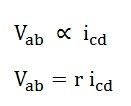# Independent Dependent Voltage and Current Source

The source which supplies the active power to the network is known as the electrical source. The electrical source is of two types namely independent source and dependent source. The Independent and Dependent source means, whether the voltage or current sources are either depending upon some other source, or they are acting independently.

There are two types of energy source direct sources and alternating sources.

Direct Source

The voltage and the current source are the direct sources. The direct source is further classified as independent voltage and current source and dependent voltage and the current source.

## Independent Voltage and Current Source

Independent sources are that which does not depend on any other quantity in the circuit. They are two-terminal devices and has a constant value, i.e. the voltage across the two terminals remains constant irrespective of all circuit conditions.

The strength of voltage or current is not changed by any variation in the connected network the source is said to be either independent voltage or independent current source. In this, the value of voltage or current is fixed and is not adjustable

## Dependent Voltage and Current Source

The sources whose output voltage or current is not fixed but depends on the voltage or current in another part of the circuit is called Dependent or Controlled source. They are four-terminal devices.

When the strength of voltage or current changes in the source for any change in the connected network, they are called dependent sources. The dependent sources are represented by a diamond shape.

The dependent sources are further categorised as:

### Voltage Controlled Voltage Source (VCVS)

In voltage-controlled voltage source, the voltage source is dependent on any element of the circuit.In the above figure, the voltage across the source terminal Vab is dependent on the voltage across the terminal Vcd,### Voltage Controlled Current Source (VCCS)

In the voltage controlled current source, the current of the source iab depends on the voltage across the terminal cd (Vcd) as shown in the figure below:

Where ƞ is a constant known as transconductance and its unit is mho.

### Current Controlled Voltage Source (CCVS)

In the current controlled voltage source voltage source of the network depends upon the current of the network as shown in the figure belowHere the voltage of source Vab depends on the current of the branch cdWhere r is a constant.

### Current Controlled Current Source (CCCS)

In the Current Controlled Current Source, the current source is dependent on the current of the branch another branch as shown in the figure below

Where β is a constant

Alternating Sources

In the network applications, there are other types of sources also where voltage or current vary with time sinusoidally or exponentially etc. are termed as alternating sources.